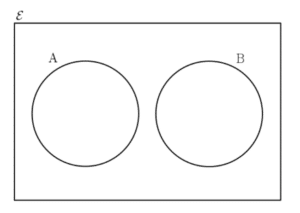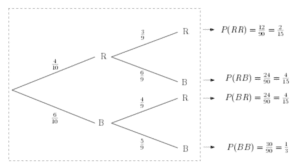# Mutually Exclusive & Independent Events

Mutually exclusive events are those that cannot happen simultaneously, whereas independent events are those whose probabilities do not affect one another. See below for more details.

## Mutually Exclusive EventsTwo events A and B are said to be mutually exclusive if it is not possible that both of them occur at the same time. For example, consider the toss of a coin. Let A be the event that the coin lands on heads and B be the event that the coin lands on tails. It follows that, in a single fair coin toss, events A and B are mutually exclusive. Mutual exclusivity can be shown on a Venn diagram (learn about Venn Diagrams). This Venn diagram shows two mutually exclusive events A and B.

It is not possible for events A and B to occur at the same time.

## Independent EventsEvents A and B are said to be independent if the probability of B occurring is unaffected by the occurrence of the event A happening. For example, now suppose that we are tossing a coin twice. Let A be the event that the first coin toss lands on heads. In addition, let B be the event that the second coin toss lands on heads. Clearly the result of the first coin toss does not affect the result of the second coin toss. See Tree Diagrams to this example in more detail. It follows that events A and B are independent.
Consider the  tree diagram shown here. The probability that event R occurs along the second branch is dependent on the outcome of the first event. It follows that events B and R are not independent. Hence, they are known as dependent events and the associated probabilities are conditional.

TBC

TBC

…coming soon.